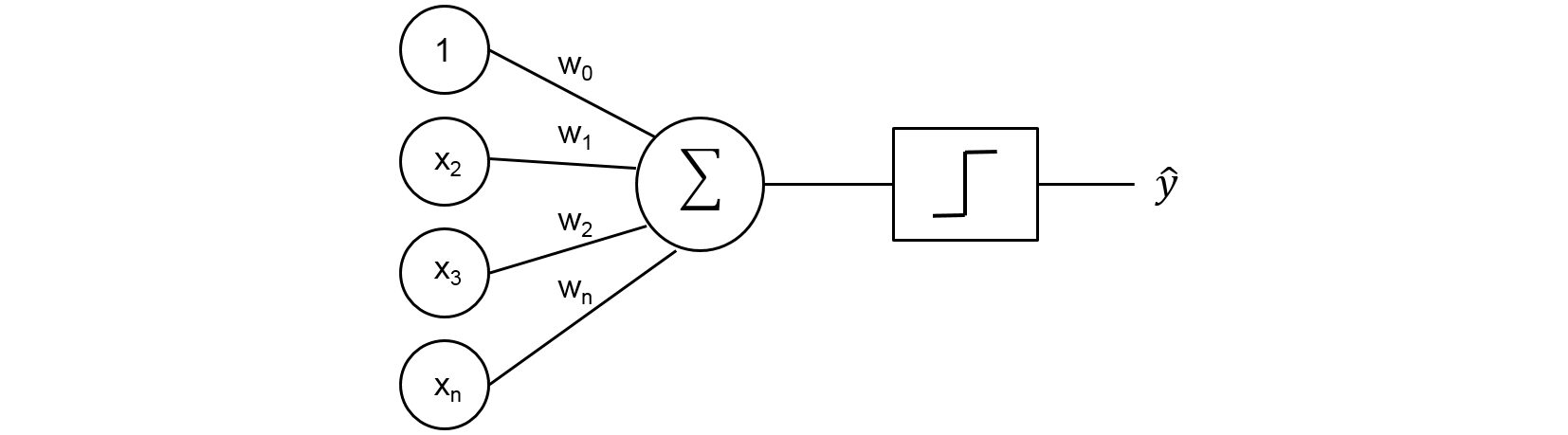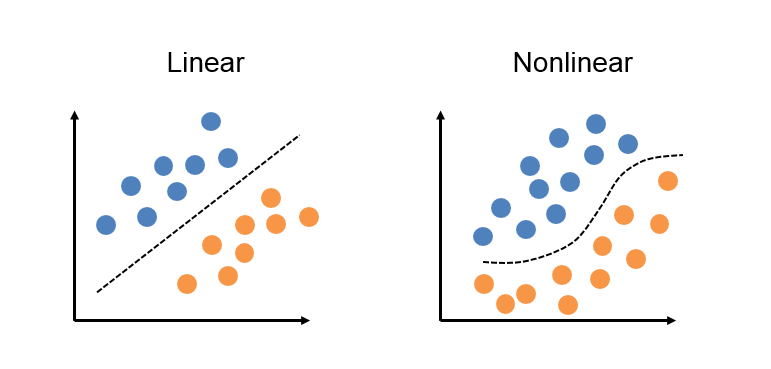# Data Wrangling Project

Here’s some basic text.

And here’s some italics

Here’s some bold text.

Here’s a bulleted list:

• First item
• Second item
• Third item

Here’s a numbered list:

1. First
2. Second
3. Third

Python code block:

    import numpy as np

def test_function(x, y):
z = np.sum(x,y)
return z


R code block:

library(tidyverse)

Here’s some inline code x+y.
Here’s an image:Here’s another image using Kramdown:You can also put it inline $z=x+y$